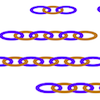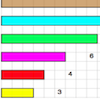# Search by Topic

Filter by: Content type:
Age range:
Challenge level:

### There are 12 results

Broad Topics > Fractions, Decimals, Percentages, Ratio and Proportion > Equivalent fractions, decimals and percentages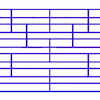### Tumbling Down

##### Age 7 to 11 Challenge Level:

Watch this animation. What do you see? Can you explain why this happens?### The Greedy Algorithm

##### Age 11 to 14 Challenge Level:

The Egyptians expressed all fractions as the sum of different unit fractions. The Greedy Algorithm might provide us with an efficient way of doing this.### Power Match

##### Age 16 to 18 Challenge Level:

Can you locate these values on this interactive logarithmic scale?### Round and Round and Round

##### Age 11 to 14 Challenge Level:

Where will the point stop after it has turned through 30 000 degrees? I took out my calculator and typed 30 000 ÷ 360. How did this help?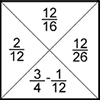### Fractions Jigsaw

##### Age 11 to 14 Challenge Level:

A jigsaw where pieces only go together if the fractions are equivalent.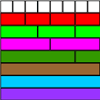### Fractional Wall

##### Age 7 to 11 Challenge Level:

Using the picture of the fraction wall, can you find equivalent fractions?### Fractions and Percentages Card Game

##### Age 11 to 16 Challenge Level:

Match the cards of the same value.### Sept 03

##### Age 11 to 14 Challenge Level:

What is the last digit of the number 1 / 5^903 ?### Repetitiously

##### Age 11 to 14 Challenge Level:

The number 2.525252525252.... can be written as a fraction. What is the sum of the denominator and numerator?### Tiny Nines

##### Age 14 to 16 Challenge Level:

Find the decimal equivalents of the fractions one ninth, one ninety ninth, one nine hundred and ninety ninth etc. Explain the pattern you get and generalise.